×#### Thank you for registering.

One of our academic counsellors will contact you within 1 working day.

Click to Chat

1800-1023-196

+91-120-4616500

CART 0

• 0

MY CART (5)

Use Coupon: CART20 and get 20% off on all online Study Material

ITEM
DETAILS
MRP
DISCOUNT
FINAL PRICE
Total Price: Rs.

There are no items in this cart.
Continue Shopping• Complete JEE Main/Advanced Course and Test Series
• OFFERED PRICE: Rs. 15,900
• View Details

```Mensuration III (Surface Area and Volume of a Right Circular Cylinder) Exercise 22.2

Question: 1

Find the volume of a cylinder whose:  (i) r = 3.5 cm, h = 40 cm (ii) r = 2.81 m, h = 15 m

Solution:Question: 2

Find the volume of a cylinder, if the diameter (d) of its base and its altitude (h) are:  (i) d = 21 cm, h = 10 cm (ii) d =7 m, h = 24 m

Solution:Question: 3

The area of the base of a right circular cylinder is 616 cm2 and its height is 25 cm. Find the volume of the cylinder.

Solution:Question: 4

The circumference of the base of a cylinder is 88 cm and its height is 15 cm. Find the volume of the cylinder.

Solution:Question: 5

A hollow cylindrical pipe is 21 mm long. Its outer and inner diameters are 10 cm and 6 cm respectively. Find the volume of the copper used in making the pipe.

Solution:Question: 6

Find the (i) curved surface area (ii) total surface area and (iii) volume of a right circular cylinder whose height is 15 cm and the radius of the base is 7 cm.

Solution:Question: 7

The diameter of the base of a right circular cylinder is 42 cm and its height is 10 cm. Find the volume of the cylinder.

Solution: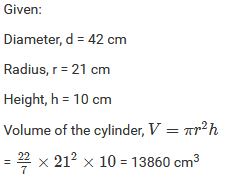Question: 8

Find the volume of a cylinder, the diameter of whose base is 7 cm and height being 60 cm. Also, find the capacity of the cylinder in liters.

Solution: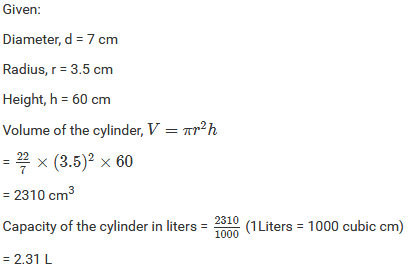Question: 9

A rectangular strip 25 cm x 7 cm is rotated on the longer side. Find the volume of the solid, thus generated.

Solution:Question: 10

A rectangular sheet of paper, 44 cm x 20 cm, is rolled along its length to form a cylinder. Find the volume of the cylinder so formed.

Solution:Question: 11

The volume and the curved surface area of a cylinder are 1650 cm3 and 660 cm2 respectively. Find the radius and height of the cylinder.

Solution:Question: 12

The radii of two cylinders are in the ratio 2 : 3 and their heights are in the ratio 5 : 3. Calculate the ratio of their volumes.

Solution: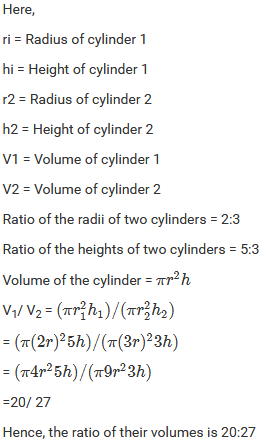Question: 13

The ratio between the curved surface area and the total surface area of a right circular cylinder is 1:2. Find the volume of the cylinder, if its total surface area is 616 cm2.

Solution:Question: 14

The curved surface area of a cylinder is 1320 cm2 and its base has diameter 21 cm. Find the volume of the cylinder.

Solution:Question: 15

The ratio between the radius of the base and the height of a cylinder is 2 : 3. Find the total surface area of the cylinder, if its volume is 1617 cm3.

Solution:Question: 16

The curved surface area of a cylindrical pillar is 264 m3 and its volume is 924 m3. Find the diameter and the height of the pillar.

Solution:Question: 17

Two circular cylinders of equal volumes have their heights in the ratio 1 : 2. Find the ratio of their radii.

Solution:Question: 18

The height of a right circular cylinder is 10.5 m. Three times the sum of the areas of its two circular faces is twice the area of the curved surface. Find the volume of the cylinder.

Solution: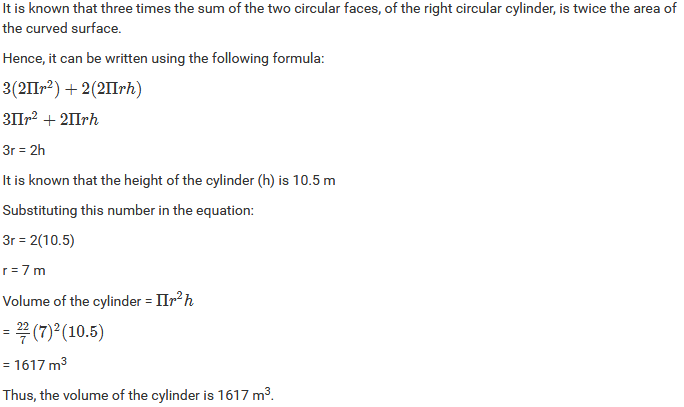Question: 19

How many cubic meters of earth must be dug-out to sink a well 21 m deep and 6 m diameter?

Solution:

The volume of the earth that must be dug out is similar to the volume of the cylinder which is equal to πr2h.

Height of the well =21 m

Diameter of the well 6 m

∴ Volume of the earth that must be dug out = (π(32)(21)) m3= 594 m3

Question: 20

The trunk of a tree is cylindrical and its circumference is 176 cm. if the length of the trunk is 3 m, find the volume of the timber that can be obtained from the trunk

Solution:Question: 21

A well is dug 20 m deep and it has a diameter of 7 m. The earth which is so dug out is spread out on a rectangular plot 22 m long and 14 m broad. What is the height of the platform so formed?

Solution:Question: 22

A well with 14 m diametre is dug 8 m deep. The earth taken out of it has been evenly spread all around it to a width of 21 m to form an embankment. Find the height of the embankment.

Solution:Question: 23

A cylindrical container with the diameter of base 56 cm contains sufficient water to submerge a rectangular solid of iron with dimension 32 cm x 22 cm x 14 cm. Find the rise in the level of the water when the solid is completely submerged.

Solution:Question: 24

A rectangle sheet of paper 30 cm x 18 cm can be transformed into the curved surface of a right circular cylinder in two ways i.e. either by rolling the paper along its length or by rolling it along its breadth. Find the ratio of the volumes of the two cylinders thus formed.

Solution:Question: 25

The rain which falls on a roof 18 m long and 16.5 m wide is allowed to be stored in a cylindrical tank 8 m in diameter. If it rains 10 cm on a day, what is the rise of water level in the tank due to it?

Solution:Question: 26

A piece of ductile metal is in the form of a cylinder of diameter 1 cm and length 5 cm. It is drawn out into a wire of diameter 1 mm. What will be the length of the wire so formed?

Solution:Question: 27

Find the length of 13.2 kg of copper wire of diameter 4 mm, when 1 cubic cm of copper weighs 8.4 gm.

Solution: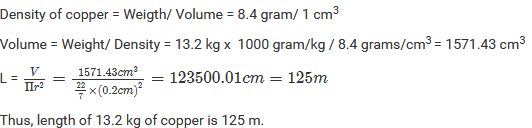Question: 28

2.2 cubic dm of brass is to be drawn into a cylindrical wire 0.25 cm in diameter. Find the length of the wire.

Solution:Question: 29

The difference between inside and outside surfaces of a cylindrical tube 14 cm long is 88 sq. cm. If the volume of the tube is 176 cubic cm, find the inner and outer radii of the tube.

Solution: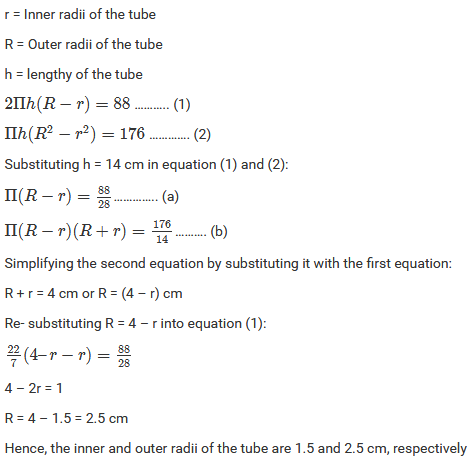Question: 30

Water flows out through a circular pipe whose internal diameter is 2 cm, at the rate of 6 meters per second into a cylindrical tank, the radius of whose base is 60 cm. Find the rise in the level of water in 30 minutes?

Solution:Question: 31

A cylindrical tube, open at both ends, is made of metal. The internal diameter of the tube is 10.4 cm and its length is 25 cm. The thickness of the metal is 8 mm everywhere. Calculate the volume of the metal.

Solution:Question: 32

From a tap of inner radius 0.75 cm, water flows at the rate of 7 m per second. Find the volume in liters of water delivered by the pipe in one hour.

Solution: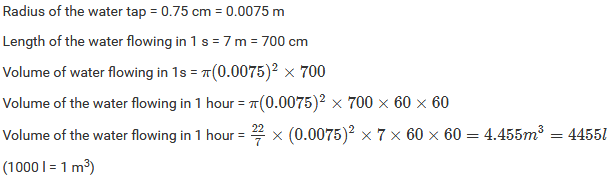Question: 33

A cylindrical water tank of diameter 1.4 m and height 2.1 m is being fed by a pipe of diameter 3.5 cm through which water flows at the rate of 2 meters per second. In how much time the tank will be filled?

Solution:Question: 34

A rectangular sheet of paper 30 cm x 18 cm can be transformed into the curved surface of a right circular cylinder in two ways i.e., either by rolling the paper along its length or by rolling it along its breadth. Find the ratio of the volumes of the two cylinders thus formed.

Solution:Question: 35

How many liters of water flow out of a pipe having an area of the cross-section of 5 cm2 in one minute, if the speed of water in the pipe is 30 cm/sec?

Solution:

We know:

Area of cross section = 5 cm2

Rate = 30 cm/s and

Time = 1 min

So, the volume of water flow is:

Volume = volumetric rate x Time = (30 cm/s)(5 cm2)(60 s/min) = 9000 cm3 = 9 litres

Thus, 9 litres of water flows out of the pipe

Question: 36

A solid cylinder has a total surface area of 231 cm2. Its curved surface area is 3 — of the total surface area. Find the volume of the cylinder.

Solution:

We know that the total surface area of the cylinder is 231 cm3 and the curved surface area of the cylinder is 2/3 of the total surface area.

So, the curved surface area is:Question: 37

Find the cost of sinking a tubewell 280 m deep, having diameter 3 m at the rate of Rs 3.60 per cubic meter. Find also the cost of cementing its inner curved surface at Rs 2.50 per square meter.

Solution:Question: 38

Find the length of 13.2 kg of copper wire of diameter 4 mm, when 1 cubic cm of copper weighs 8.4 gm.

Solution:Question: 39

2.2 cubic dm of brass is to be drawn into a cylindrical wire 0.25 cm in diameter. Find the length of the wire.

Solution:Question: 40

A well with 10 in inside diameter is dug 8.4 m deep. Earth taken out of it is spread all around it to a width of 7.5 m to form an embankment. Find the height of the embankment.

Solution: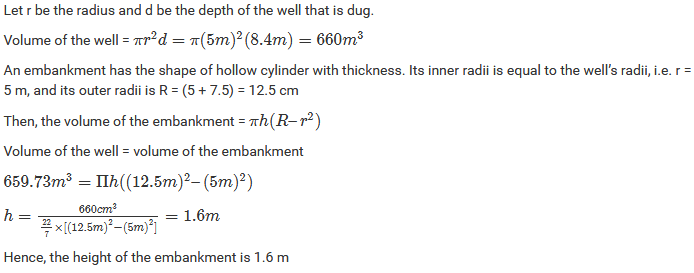Question: 41

A hollow garden roller, 63 cm wide with a girth of 440 cm, is made of 4 cm thick iron. Find the volume of the iron.

Solution:Question: 42

What length of a solid cylinder 2 cm in diameter must be taken to recast into a hollow cylinder of length 16 cm, external diameter 20 cm and thickness 2.5 mm?

Solution:Question: 43

In the middle of a rectangular field measuring 30m x 20m, a well of 7 m diameter and 10 m of depth is dug. The earth so removed is evenly spread over the remaining part the field. Find the height through which the level of the field is raised.

Solution:```### Course Features

• 728 Video Lectures
• Revision Notes
• Previous Year Papers
• Mind Map
• Study Planner
• NCERT Solutions
• Discussion Forum
• Test paper with Video Solution